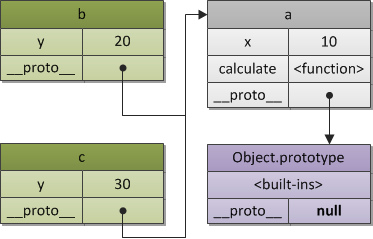036 编程范式游记（7）- 基于原型的编程范式

• 在基于类的编程当中，对象总共有两种类型。类定义了对象的基本布局和函数特性，而接口是“可以使用的”对象，它基于特定类的样式。在此模型中，类表现为行为和结构的集合，对所有接口来说这些类的行为和结构都是相同的。因而，区分规则首先是基于行为和结构，而后才是状态。
• 原型编程的主张者经常争论说，基于类的语言提倡使用一个关注分类和类之间关系的开发模型。与此相对，原型编程看起来提倡程序员关注一系列对象实例的行为，而之后才关心如何将这些对象划分到最近的使用方式相似的原型对象，而不是分成类。

• 在基于类的语言中，一个新的实例通过类构造器和构造器可选的参数来构造，结果实例由类选定的行为和布局创建模型。
• 在基于原型的系统中构造对象有两种方法，通过复制已有的对象或者通过扩展空对象创建。很多基于原型的系统提倡运行时原型的修改，而基于类的面向对象系统只有动态语言允许类在运行时被修改（Common Lisp、Dylan、Objective-C、Perl、Python、Ruby 和 Smalltalk）。

JavaScript 的原型概念

var foo = {name: "foo", one: 1, two: 2};

var bar = {three: 3};

bar.__proto__ = foo; // foo is now the prototype of bar.

// If we try to access foo's properties from bar
// from now on, we'll succeed.
bar.one // Resolves to 1.

// The child object's properties are also accessible.
bar.three // Resolves to 3.

// Own properties shadow prototype properties
bar.name = "bar";
foo.name; // unaffected, resolves to "foo"
bar.name; // Resolves to "bar"

• proto 主要是安放在一个实际的对象中，用它来产生一个链接，一个原型链连，用于寻找方法名或属性，等等。
• prototype 是用 new 来创建一个对象时构造 __proto__ 用的。它是构造函数的一个属性。

var a = {
x: 10,
calculate: function (z) {
return this.x + this.y + z;
}
};

var b = {
y: 20,
__proto__: a
};

var c = {
y: 30,
__proto__: a
};

// call the inherited method
b.calculate(30); // 60
c.calculate(40); // 80var b = Object.create(a, {y: {value: 20}});
var c = Object.create(a, {y: {value: 30}});

// 一种构造函数写法
function Foo(y) {
this.y = y;
}

// 修改 Foo 的 prototype，加入一个成员变量 x
Foo.prototype.x = 10;

// 修改 Foo 的 prototype，加入一个成员函数 calculate
Foo.prototype.calculate = function (z) {
return this.x + this.y + z;
};

// 现在，我们用 Foo 这个原型来创建 b 和 c
var b = new Foo(20);
var c = new Foo(30);

// 调用原型中的方法，可以得到正确的值
b.calculate(30); // 60
c.calculate(40); // 80b.__proto__ === Foo.prototype, // true
c.__proto__ === Foo.prototype, // true

b.constructor === Foo, // true
c.constructor === Foo, // true
Foo.prototype.constructor === Foo, // true

b.calculate === b.__proto__.calculate, // true
b.__proto__.calculate === Foo.prototype.calculate // true

Foo.prototype 自动创建了一个属性 constructor，这是一个指向函数自己的一个 reference。这样一来，对于实例 b 或 c 来说，就能访问到这个继承的 constructor 了。

JavaScript 原型编程的面向对象

function Person(){}
var p = new Person();

Person.prototype.name = "Hao Chen";
Person.prototype.sayHello = function(){
console.log("Hi, I am " + this.name);
}

console.log(p.name); // "Hao Chen"
p.sayHello(); // "Hi, I am Hao Chen"

• 我们先生成了一个空的函数对象 Person()
• 然后将这个空的函数对象 new 出另一个对象，存在 p 中；
• 这时再改变 Person.prototype，让其有一个 name 的属性和一个 sayHello() 的方法；
• 我们发现，另外那个 p 的对象也跟着一起改变了。

• 当创建 function Person(){} 时，Person.__proto__ 指向 Function.prototype;
• 当创建 var p = new Person() 时，p.__proto__ 指向 Person.prototype;
• 当修改了 Person.prototype 的内容后，p.__proto__ 的内容也就被改变了。

//Define human class
var Person = function (fullName, email) {
this.fullName = fullName;
this.email = email;

this.speak = function(){
console.log("I speak English!");
};
this.introduction = function(){
console.log("Hi, I am " + this.fullName);
};
}

• 属性： fullNameemail
• 方法： speak()introduction()

//Define Student class
var Student = function(fullName, email, school, courses) {

Person.call(this, fullName, email);

// Initialize our Student properties
this.school = school;
this.courses = courses;

// override the "introduction" method
this.introduction= function(){
console.log("Hi, I am " + this.fullName +
". I am a student of " + this.school +
", I study "+ this.courses +".");
};

this.takeExams = function(){
console.log("This is my exams time!");
};
};

• 使用了 Person.call(this, fullName, email)call()apply() 都是为了动态改变 this 所指向的对象的内容而出现的。这里的 this 就是 Student
• 上面的例子中，我们重载了 introduction() 方法，并新增加了一个 takeExams()的方法。

• 先用 Object.create() 来将Person.prototypeStudent.prototype 关联上。
• 然后，修改一下构造函数 Student.prototype.constructor = Student;
// Create a Student.prototype object that inherits
// from Person.prototype.
Student.prototype = Object.create(Person.prototype);

// Set the "constructor" property to refer to Student
Student.prototype.constructor = Student;

var student = new Student("Hao Chen",
"[email protected]",
"XYZ University",
"Computer Science");
student.introduction();
student.speak();
student.takeExams();

// Check that instanceof works correctly
console.log(student instanceof Person);  // true
console.log(student instanceof Student); // true